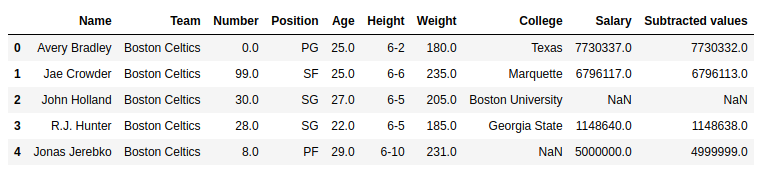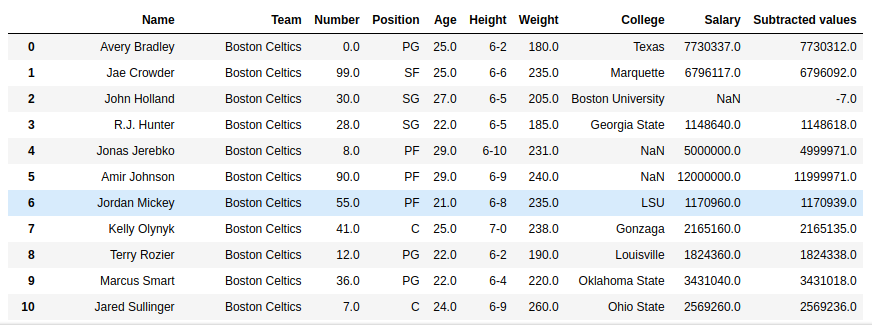Skip to content
Related Articles
Python | Pandas Series.sub()
• Last Updated : 01 Oct, 2018

Python is a great language for doing data analysis, primarily because of the fantastic ecosystem of data-centric Python packages. Pandas is one of those packages and makes importing and analyzing data much easier.

Python `Series.sub()` is used to subtract series or list like objects with same length from the caller series.

Syntax: Series.sub(other, level=None, fill_value=None, axis=0)

Parameters:
other: other series or list type to be subtracted from caller series
fill_value: Value to be replaced by NaN in series/list before subtracting
level: integer value of level in case of multi index

Return type: Caller series with subtracted values

To download the data set used in following example, click here.
In the following examples, the data frame used contains data of some NBA players. The image of data frame before any operations is attached below.Example #1: Subtracting List

In this example, the top 5 rows are stored in new variable using .head() method. After that a list of same length is created and subtracted from the salary column using .sub() method

 `# importing pandas module ``import` `pandas as pd`` ` `# reading csv file from url ``data ``=` `pd.read_csv(``"https://media.geeksforgeeks.org/wp-content/uploads/nba.csv"``)`` ` `# creating short data of 5 rows``short_data ``=` `data.head()`` ` `# creating list with 5 values``list` `=``[``5``, ``4``, ``3``, ``2``, ``1``]`` ` `# subtracting list data``# creating new column``short_data[``"Subtracted values"``]``=` `short_data[``"Salary"``].sub(``list``)`` ` `# display``short_data`

Output:
As shown in the output image, it can be compared that the Subtracted values column is having the subtracted values of Salary column – list.Example #2: Adding series to series with null values

In this example, the Age column is subtracted from the Salary column. Since the salary column contains null values too, by default it returns NaN no matter what is subtracted. In this example, 20 is passed to replace null values with 20.

 `# importing pandas module ``import` `pandas as pd`` ` `# reading csv file from url ``data ``=` `pd.read_csv(``"https://media.geeksforgeeks.org/wp-content/uploads/nba.csv"``)`` ` `# age series``age ``=` `data[``"Age"``]`` ` `# na replacement``na ``=` `20`` ` `# adding values``# storing to new column``data[``"Subtracted values"``]``=` `data[``"Salary"``].sub(other ``=` `age, fill_value ``=` `na)`` ` `# display``data`

Output:
As shown in the output image, the Subtracted value column has subtracted age column from 20 in case of Null values.Attention geek! Strengthen your foundations with the Python Programming Foundation Course and learn the basics.

To begin with, your interview preparations Enhance your Data Structures concepts with the Python DS Course. And to begin with your Machine Learning Journey, join the Machine Learning – Basic Level Course

My Personal Notes arrow_drop_up